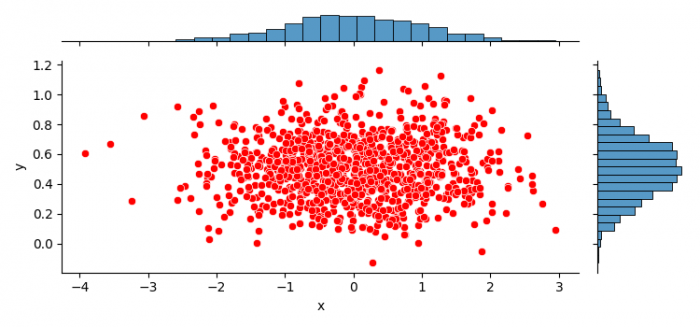# How to plot a non-square Seaborn jointplot or JointGrid? (Matplotlib)

To plot a non-square Seaborn jointplot or jointgrid, we can use set_figwidth() and set_figheight() methods.

## Steps

• Set the figure size and adjust the padding between and around the subplots.
• Create x and y data points using numpy.
• Create a dataframe with two columns.
• Use jointplot() method to plot the jointplot.
• To make it non-square, we can set the figure width and height.
• To display the figure, use show() method.

## Example

import seaborn as sns
import numpy as np
from matplotlib import pyplot as plt
import pandas as pd

plt.rcParams["figure.figsize"] = [7.50, 3.50]
plt.rcParams["figure.autolayout"] = True

X = np.random.randn(1000,)
Y = 0.2 * np.random.randn(1000) + 0.5

df = pd.DataFrame(dict(x=X, y=Y))

jp = sns.jointplot(x="x", y="y", data=df, height=3.5,
joint_kws={'color': 'red'})
jp.fig.set_figwidth(7.50)
jp.fig.set_figheight(3.50)

plt.show()

## Output